### Python Lab Tutorials: Lab 2

1. Consider the relationship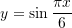Using Sympy, find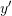and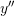. Evaluate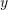,, andat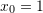. Then, using Matplotlib, plot the relationships,, andfor the domain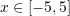by first finding the y-values using a “for” loop and a list. Label your plot and plot legend appropriately.
2. Consider the sequence whose terms are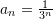. Use Sympy to evaluate the sum of the first twenty terms. Then, evaluate the infinite sum: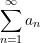Use Matplotlib to plot the first ten terms of the ordered pairs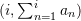. Label the plot appropriately.
3. Consider the matrix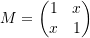Use Matplotlib and Sympy to plot the relationship between the determinant of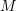andfor. Label the plot appropriately. From the plot, can you identify the values offor which the determinant is equal to zero?
4. The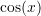function whereis in radian can be represented as the infinite series: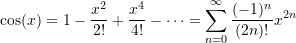Let i+1 be the number of terms used to approximate the function. Using Numpy, create a function in Python whose inputs areandand whose output is the approximation: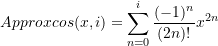Show using numerical examples that your function gives proper approximations by comparing its output with the built-infunction in Numpy.

## Extra Practice Problems

1. The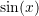function whereis in radian can be represented as the infinite series: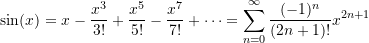Let i+1 be the number of terms used to approximate the function. Using Numpy, create a function in Python whose inputs areandand whose output is the approximation: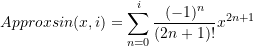Show using numerical examples that your function gives proper approximations by comparing its output with the built-in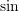function in Numpy.
2. The discharge velocity through an orifice at the bottom of a water tank open to the atmosphere is given by the relationship: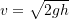where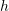is the height of the water left in the tank. If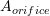is the “effective” area of the orifice and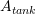is the cross sectional area of the tank, then the following differential equation describes the rate of change of the height of the water left in the tank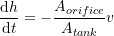Assuming that at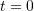the height of the water in the tank is given by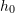, use the Sympy’s dsolve function to solve the above differential equation using Python to find the heightas a function of,,,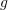, and. Then, use the “Solve” function to show that the time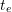to empty the tank (i.e., the time at which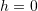) is given by the expression: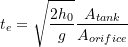3. In Sympy or Numpy, write two functions in Python whose arguments are a matrix and a vector. The first function should output the matrix after replacing the last row with the given vector. The second function should output the matrix after replacing the last column with the given vector.